January 22, 2017

# Neon Hair Battle: Sunset Gladiator

VIEW ALL PHOTOS VIEW SLIDESHOW
GET THE HOW-TO• Formula A

Kenra Professional Simply Blonde Blue Powder Lightener + 20-volume Simply Blonde Developer

• Formula B

Kenra Professional Demi Permanent 7VM + 9-volume developer

• Formula C

Demi Permanent 10SM + 9-volume developer

• Formula D

Demi Permanent 5VR + 2 inches Blue Booster + 9-volume developer

• Formula E

Demi Permanent 6RR + 9-volume developer

• Formula F

Creative Red

• Formula G

Neon Orange

• Formula H

Creative Yellow

• Formula I

Creative Pink

• Formula J

Neon Violet

• Formula K

Neon Blue

• Formula L

Creative Blue# Neon Hair Battle: Sunset Gladiator

Megan Schipani (@shmeggsandbaconn) brought her A-game to LA as one of the five finalists in the Kenra Professional 2017 Neon Hair Battle, and it was an epic weekend-long neon throwdown. Megan went for a dramatic look by toning with metallics before applying her creative and neon shades. Find out how she achieved this Sunset Gladiator!

See the Kenra team onstage Aug. 20-22 at our COLOR, Cut & Style show in Austin, Texas! TICKETS ARE NOW ON SALE!

## COLOR FORMULAS• Formula A

Kenra Professional Simply Blonde Blue Powder Lightener + 20-volume Simply Blonde Developer

• Formula B

Kenra Professional Demi Permanent 7VM + 9-volume developer

• Formula C

Demi Permanent 10SM + 9-volume developer

• Formula D

Demi Permanent 5VR + 2 inches Blue Booster + 9-volume developer

• Formula E

Demi Permanent 6RR + 9-volume developer

• Formula F

Creative Red

• Formula G

Neon Orange

• Formula H

Creative Yellow

• Formula I

Creative Pink

• Formula J

Neon Violet

• Formula K

Neon Blue

• Formula L

Creative Blue

## HOW-TO STEPS•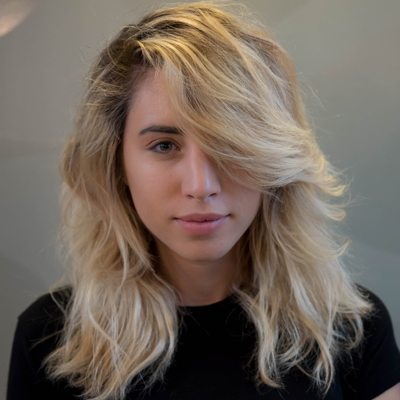• Formula A

Kenra Professional Simply Blonde Blue Powder Lightener + 20-volume Simply Blonde Developer

• Formula B

Kenra Professional Demi Permanent 7VM + 9-volume developer

• Formula C

Demi Permanent 10SM + 9-volume developer

• Formula D

Demi Permanent 5VR + 2 inches Blue Booster + 9-volume developer

• Formula E

Demi Permanent 6RR + 9-volume developer

• Formula F

Creative Red

• Formula G

Neon Orange

• Formula H

Creative Yellow

• Formula I

Creative Pink

• Formula J

Neon Violet

• Formula K

Neon Blue

• Formula L

Creative Blue

1

Before

•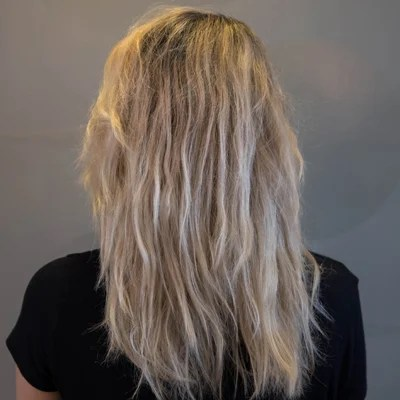• Formula A

Kenra Professional Simply Blonde Blue Powder Lightener + 20-volume Simply Blonde Developer

• Formula B

Kenra Professional Demi Permanent 7VM + 9-volume developer

• Formula C

Demi Permanent 10SM + 9-volume developer

• Formula D

Demi Permanent 5VR + 2 inches Blue Booster + 9-volume developer

• Formula E

Demi Permanent 6RR + 9-volume developer

• Formula F

Creative Red

• Formula G

Neon Orange

• Formula H

Creative Yellow

• Formula I

Creative Pink

• Formula J

Neon Violet

• Formula K

Neon Blue

• Formula L

Creative Blue

2

Before – back view

•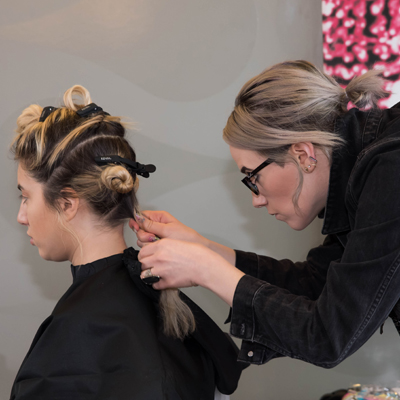• Formula A

Kenra Professional Simply Blonde Blue Powder Lightener + 20-volume Simply Blonde Developer

• Formula B

Kenra Professional Demi Permanent 7VM + 9-volume developer

• Formula C

Demi Permanent 10SM + 9-volume developer

• Formula D

Demi Permanent 5VR + 2 inches Blue Booster + 9-volume developer

• Formula E

Demi Permanent 6RR + 9-volume developer

• Formula F

Creative Red

• Formula G

Neon Orange

• Formula H

Creative Yellow

• Formula I

Creative Pink

• Formula J

Neon Violet

• Formula K

Neon Blue

• Formula L

Creative Blue

3

Lighten dark bands with Formula A.

•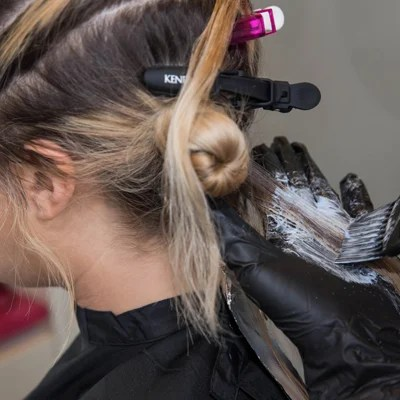• Formula A

Kenra Professional Simply Blonde Blue Powder Lightener + 20-volume Simply Blonde Developer

• Formula B

Kenra Professional Demi Permanent 7VM + 9-volume developer

• Formula C

Demi Permanent 10SM + 9-volume developer

• Formula D

Demi Permanent 5VR + 2 inches Blue Booster + 9-volume developer

• Formula E

Demi Permanent 6RR + 9-volume developer

• Formula F

Creative Red

• Formula G

Neon Orange

• Formula H

Creative Yellow

• Formula I

Creative Pink

• Formula J

Neon Violet

• Formula K

Neon Blue

• Formula L

Creative Blue

4

Tone the roots with Formula B and the ends with Formula C.

•• Formula A

Kenra Professional Simply Blonde Blue Powder Lightener + 20-volume Simply Blonde Developer

• Formula B

Kenra Professional Demi Permanent 7VM + 9-volume developer

• Formula C

Demi Permanent 10SM + 9-volume developer

• Formula D

Demi Permanent 5VR + 2 inches Blue Booster + 9-volume developer

• Formula E

Demi Permanent 6RR + 9-volume developer

• Formula F

Creative Red

• Formula G

Neon Orange

• Formula H

Creative Yellow

• Formula I

Creative Pink

• Formula J

Neon Violet

• Formula K

Neon Blue

• Formula L

Creative Blue

5

Split the hair into two sections along the occipital. Apply Formula D to the roots in the top section and apply Formula E to the roots in the bottom section.

•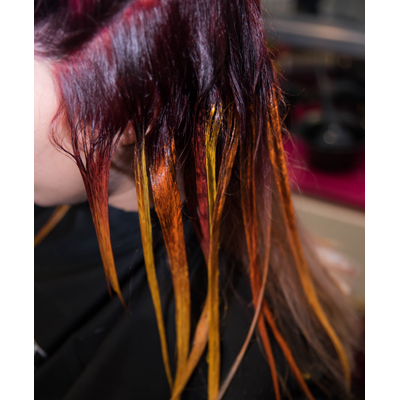• Formula A

Kenra Professional Simply Blonde Blue Powder Lightener + 20-volume Simply Blonde Developer

• Formula B

Kenra Professional Demi Permanent 7VM + 9-volume developer

• Formula C

Demi Permanent 10SM + 9-volume developer

• Formula D

Demi Permanent 5VR + 2 inches Blue Booster + 9-volume developer

• Formula E

Demi Permanent 6RR + 9-volume developer

• Formula F

Creative Red

• Formula G

Neon Orange

• Formula H

Creative Yellow

• Formula I

Creative Pink

• Formula J

Neon Violet

• Formula K

Neon Blue

• Formula L

Creative Blue

6

On the bottom section, melt Formula F into Formula G, then melt into Formula H.

•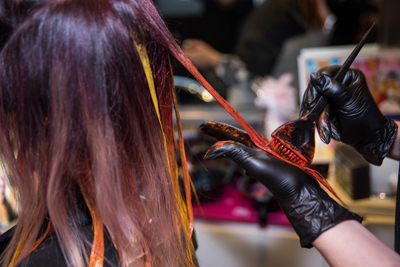• Formula A

Kenra Professional Simply Blonde Blue Powder Lightener + 20-volume Simply Blonde Developer

• Formula B

Kenra Professional Demi Permanent 7VM + 9-volume developer

• Formula C

Demi Permanent 10SM + 9-volume developer

• Formula D

Demi Permanent 5VR + 2 inches Blue Booster + 9-volume developer

• Formula E

Demi Permanent 6RR + 9-volume developer

• Formula F

Creative Red

• Formula G

Neon Orange

• Formula H

Creative Yellow

• Formula I

Creative Pink

• Formula J

Neon Violet

• Formula K

Neon Blue

• Formula L

Creative Blue

7

On the top section, melt Formula I into Formula J on some sections and melt Formula K into Formula J on other sections.

•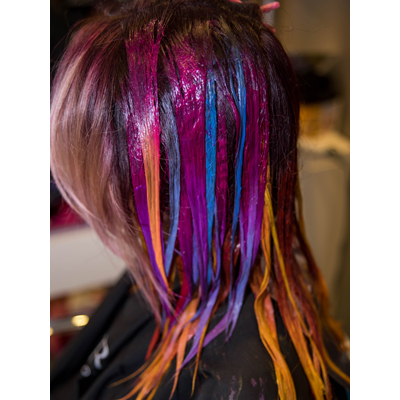• Formula A

Kenra Professional Simply Blonde Blue Powder Lightener + 20-volume Simply Blonde Developer

• Formula B

Kenra Professional Demi Permanent 7VM + 9-volume developer

• Formula C

Demi Permanent 10SM + 9-volume developer

• Formula D

Demi Permanent 5VR + 2 inches Blue Booster + 9-volume developer

• Formula E

Demi Permanent 6RR + 9-volume developer

• Formula F

Creative Red

• Formula G

Neon Orange

• Formula H

Creative Yellow

• Formula I

Creative Pink

• Formula J

Neon Violet

• Formula K

Neon Blue

• Formula L

Creative Blue

8

Create a peekaboo triangle-shaped section at the nape and alternate between Formulas L, J and K.

•• Formula A

Kenra Professional Simply Blonde Blue Powder Lightener + 20-volume Simply Blonde Developer

• Formula B

Kenra Professional Demi Permanent 7VM + 9-volume developer

• Formula C

Demi Permanent 10SM + 9-volume developer

• Formula D

Demi Permanent 5VR + 2 inches Blue Booster + 9-volume developer

• Formula E

Demi Permanent 6RR + 9-volume developer

• Formula F

Creative Red

• Formula G

Neon Orange

• Formula H

Creative Yellow

• Formula I

Creative Pink

• Formula J

Neon Violet

• Formula K

Neon Blue

• Formula L

Creative Blue

9

Finished look.

•• Formula A

Kenra Professional Simply Blonde Blue Powder Lightener + 20-volume Simply Blonde Developer

• Formula B

Kenra Professional Demi Permanent 7VM + 9-volume developer

• Formula C

Demi Permanent 10SM + 9-volume developer

• Formula D

Demi Permanent 5VR + 2 inches Blue Booster + 9-volume developer

• Formula E

Demi Permanent 6RR + 9-volume developer

• Formula F

Creative Red

• Formula G

Neon Orange

• Formula H

Creative Yellow

• Formula I

Creative Pink

• Formula J

Neon Violet

• Formula K

Neon Blue

• Formula L

Creative Blue

10

Finished look – front

•• Formula A

Kenra Professional Simply Blonde Blue Powder Lightener + 20-volume Simply Blonde Developer

• Formula B

Kenra Professional Demi Permanent 7VM + 9-volume developer

• Formula C

Demi Permanent 10SM + 9-volume developer

• Formula D

Demi Permanent 5VR + 2 inches Blue Booster + 9-volume developer

• Formula E

Demi Permanent 6RR + 9-volume developer

• Formula F

Creative Red

• Formula G

Neon Orange

• Formula H

Creative Yellow

• Formula I

Creative Pink

• Formula J

Neon Violet

• Formula K

Neon Blue

• Formula L

Creative Blue

11

Natural light – back view

•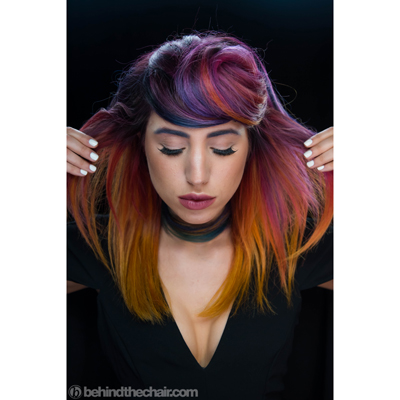• Formula A

Kenra Professional Simply Blonde Blue Powder Lightener + 20-volume Simply Blonde Developer

• Formula B

Kenra Professional Demi Permanent 7VM + 9-volume developer

• Formula C

Demi Permanent 10SM + 9-volume developer

• Formula D

Demi Permanent 5VR + 2 inches Blue Booster + 9-volume developer

• Formula E

Demi Permanent 6RR + 9-volume developer

• Formula F

Creative Red

• Formula G

Neon Orange

• Formula H

Creative Yellow

• Formula I

Creative Pink

• Formula J

Neon Violet

• Formula K

Neon Blue

• Formula L

Creative Blue

12

Natural light – front view